# Each day High and Low Values of Index indicator.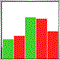540

Hello,
I am working on an index indicator based from this one :
https://www.mql5.com/ru/code/9780

I really need to get the HIGH and LOW's values (from the subwindow) of each day then i will be able to draw boxes (with OBJ_RECTANGLE).
I know how to get it from the price's chart to draw boxes on the cross, but i want to do the same on the indicator (in the subwindow), then i can compare cross boxes with indexes boxes.

Heres is the result i want to get (subwindow indicator's boxes are drawn by hand) :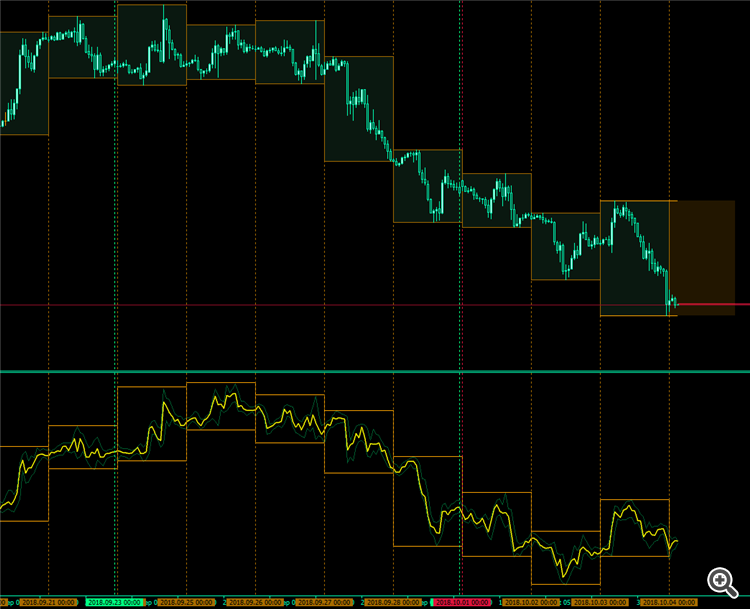To get the high and low from the chart is now easy for me, something like this :

```for(int id=0;id<Days_To_Process;id++)
{
tname=TimeToString(iTime(NULL,PERIOD_D1,id+1));
t_Start=iTime(NULL,PERIOD_D1,id+1);
t_End=iTime(NULL,PERIOD_D1,id+0);
high=iHigh(NULL,PERIOD_D1,id+1);
low=iLow(NULL,PERIOD_D1,id+1);
open=iOpen(NULL,PERIOD_D1,id+1);
close=iClose(NULL,PERIOD_D1,id+1);

// ...etc

}
// It gets the high and low of each day from the chart's price.
// But how to get the "iHigh" and "iLow" 's value from the subwindow ?```

Kind regards.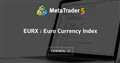EURX : Euro Currency Index
• www.mql5.com
Данный индикатор вычисляет и показывает индекс евро и скользящую и экспоненциальную средние. Для того, чтобы индикатор работал, нужно чтобы брокер предоставлял котировки по парам EURUSD, EURGBP, EURJPY, EURCHF, EURSEK. Код для индикатора взят из http://codebase.mql4.com/ru/code/9397 . Формулы для расчета взяты из...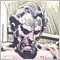3064

Thierry Ramaniraka:

Hello,
I am working on an index indicator based from this one :
https://www.mql5.com/ru/code/9780

I really need to get the HIGH and LOW's values (from the subwindow) of each day then i will be able to draw boxes (with OBJ_RECTANGLE).
I know how to get it from the price's chart to draw boxes on the cross, but i want to do the same on the indicator (in the subwindow), then i can compare cross boxes with indexes boxes.

Heres is the result i want to get (subwindow indicator's boxes are drawn by hand) :

To get the high and low from the chart is now easy for me, something like this :

Kind regards.

Two loops.

Pseudo code looks like this:

for(int i=pos;i<rates_total - 1 && !IsStopped();i++) . . . loops over all bars

At start of new day:

for ( int j=i-Lookback + 1; j<i+1; j++)

{

double hi = MathMax(hi,value[j]);

double lo = MathMin(lo,value[j]);

}

From your picture, it appears Lookback would be 24 as you are on the H1 chart.540

Anthony Garot:

Two loops.

Pseudo code looks like this:

for(int i=pos;i<rates_total - 1 && !IsStopped();i++) . . . loops over all bars

At start of new day:

for ( int j=i-Lookback + 1; j<i+1; j++)

{

double hi = MathMax(hi,value[j]);

double lo = MathMin(lo,value[j]);

}

From your picture, it appears Lookback would be 24 as you are on the H1 chart.

I wil take a look.
NB : my lookback is 20.

Thank you.3064

Thierry Ramaniraka:

NB : my lookback is 20.

However many bars/values you have in each box.540

Anthony Garot:

for(int i=pos;i<rates_total - 1 && !IsStopped();i++) . . . loops over all bars

At start of new day:

for ( int j=i-Lookback + 1; j<i+1; j++)

{

double hi = MathMax(hi,value[j]);

double lo = MathMin(lo,value[j]);

}

I need you to help me further very please.

If i am right, here is the idea :

```//| ---------------------------+
//| for chart's price          |
//| ---------------------------+
for(int id=0;id<Days_To_Process;id++)
{
string tname=TimeToString(iTime(NULL,PERIOD_D1,id+1));
datetime t_Start=iTime(NULL,PERIOD_D1,id+1);
datetime t_End=iTime(NULL,PERIOD_D1,id+0);

double high=iHigh(NULL,PERIOD_D1,id+1);
double low=iLow(NULL,PERIOD_D1,id+1);

// --- then i draw my rectangles on the main chart window
My_Draw_Rectangle_Function(subwindow,                             // the subwindow
"Chart_Rectangles_Day_High_Low"+tname, // the name
t_Start,                               // time1
high,                                  // price1
t_End,                                 // time2
low);                                  // price2
}

//| ---------------------------+
//| for subwindow's values     |
//| ---------------------------+
for(int id=0;id<Days_To_Process;id++)
{
string tname=TimeToString(iTime(NULL,PERIOD_D1,id+1));
datetime t_Start=iTime(NULL,PERIOD_D1,id+1);
datetime t_End=iTime(NULL,PERIOD_D1,id+0);

double high=MathMax(high,value[id]); // --- inspired by your code
double low=MathMin(low,value[id]);   // --- inspired by your code

// --- then i draw my rectangles in the subwindow
My_Draw_Rectangle_Function(subwindow,                                 // the subwindow
"Subwindow_Rectangles_Day_High_Low"+tname, // the name
t_Start,                                   // time1
high,                                      // price1
t_End,                                     // time2
low);                                      // price2
}

// Now, how can I get the "value" ?```

But, with my actual skills, i don't know how to get the "values" (high and low of the indicator for each day).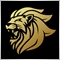Moderator
12386

Why don't you read the indicator buffers ?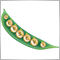6535

The easiest way is probably to use iBarShift().

```//+------------------------------------------------------------------+
//| Custom indicator iteration function                              |
//+------------------------------------------------------------------+
int OnCalculate(const int rates_total,
const int prev_calculated,
const datetime &time[],
const double &open[],
const double &high[],
const double &low[],
const double &close[],
const long &tick_volume[],
const long &volume[],
{
//---
int begin=!prev_calculated?iBarShift(_Symbol,_Period,iTime(_Symbol,PERIOD_D1,Days-2)):rates_total-prev_calculated;
int day=Days-2,shift,max,min;
for(int i=begin;i>0 && !_StopFlag;i-=1440/_Period)
{
max=min=i+1;
for(int k=(shift=iBarShift(_Symbol,_Period,iTime(_Symbol,PERIOD_D1,day+1)));k>i;k--)
{
if(high[k]>high[max])   //replace with buffer[]
max=k;
if(low[k]<low[min])     //replace with buffer[]
min=k;
}
for(int k=shift;k>i;k--)
{
HighBuffer[k]=high[max];
LowBuffer[k]=low[min];
}
day--;
}
//--- return value of prev_calculated for next call
return(rates_total);
}
//+------------------------------------------------------------------+
```3064

Thierry Ramaniraka:

But, with my actual skills, i don't know how to get the "values" (high and low of the indicator for each day).

Marco vd Heijden:
Why don't you read the indicator buffers ?540

Marco vd Heijden:
Why don't you read the indicator buffers ?
Anthony Garot:

how can it be ?
it all refers to native/primitive indicator's function in mql (like iRSI, iStochastic...etc)

Can you give me more please ?

EDIT :
maybe with this (?) : https://www.mql5.com/en/docs/series/ihighest

// ---

Ernst Van Der Merwe:

The easiest way is probably to use iBarShift().

I will take a look. It seems to be what i want.
I will try.

// ---

thank you all3064

Thierry Ramaniraka:

how can it be ?
it all refers to native/primitive indicator's function in mql (like iRSI, iStochastic...etc)

Can you give me more please ?

I don't know much about MQL4 indicators. Accessing buffers in MQL5 is pretty straightforward and logical.

This looks like it will lead you to the correct answer for MQL4.
https://www.mql5.com/en/forum/136819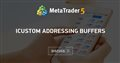• 2011.11.27
• www.mql5.com
I'm sure this has been answered before but I've searched and can not find the answer. How is a buffer value addressed/accessed through iCustom...540

Ernst Van Der Merwe:

The easiest way is probably to use iBarShift().

```
//|------------------------------------------------------+
//| Where i am so far (still need your help, very please)|
//| -----------------------------------------------------+
// --- calculation
// --- Index calculation
for(int i=limit; i>=0; i--)
{
// --- close
ExtMapBuffer1[i]=1
*MathPow(iClose("EURUSD",0,i),0.1428571428571429)
*MathPow(iClose("EURJPY",0,i),0.1428571428571429)
*MathPow(iClose("EURGBP",0,i),0.1428571428571429)
*MathPow(iClose("EURAUD",0,i),0.1428571428571429)
*MathPow(iClose("EURNZD",0,i),0.1428571428571429)
*MathPow(iClose("EURCHF",0,i),0.1428571428571429);
// --- high
ExtMapBuffer2[i]=1
*MathPow(iHigh("EURUSD",0,i),0.1428571428571429)
*MathPow(iHigh("EURJPY",0,i),0.1428571428571429)
*MathPow(iHigh("EURGBP",0,i),0.1428571428571429)
*MathPow(iHigh("EURAUD",0,i),0.1428571428571429)
*MathPow(iHigh("EURNZD",0,i),0.1428571428571429)
*MathPow(iHigh("EURCHF",0,i),0.1428571428571429);
// --- low
ExtMapBuffer3[i]=1
*MathPow(iLow("EURUSD",0,i),0.1428571428571429)
*MathPow(iLow("EURJPY",0,i),0.1428571428571429)
*MathPow(iLow("EURGBP",0,i),0.1428571428571429)
*MathPow(iLow("EURAUD",0,i),0.1428571428571429)
*MathPow(iLow("EURNZD",0,i),0.1428571428571429)
*MathPow(iLow("EURCHF",0,i),0.1428571428571429);
}

//|-----------------------------------------+
//| subwindow day ranges test from mql help |
//|-----------------------------------------+
int Days=20; // I suppose this is the "day to process" int ?

int begin=!prev_calculated?iBarShift(_Symbol,_Period,iTime(_Symbol,PERIOD_D1,Days-2)):rates_total-prev_calculated;
int day=Days-2,shift,max,min;
for(int i=begin;i>0 && !_StopFlag;i-=1440/_Period)
{
max=min=i+1;
for(int k=(shift=iBarShift(_Symbol,_Period,iTime(_Symbol,PERIOD_D1,day+1)));k>i;k--)
{
if(high[k]>high[max])   //replace with buffer[]  //|-----------------------------------------+
max=k;                                       //| Above are my calculated buffers.        |
if(low[k]<low[min])     //replace with buffer[]  //| What need to be replaced                |
min=k;                                       //| by what buffers please ?                |
}                                                  //|-----------------------------------------+
for(int k=shift;k>i;k--)
{                                                  //|-----------------------------------------+
HighBuffer[k]=high[max];                         //| And here too. I move forward with your  |
LowBuffer[k]=low[min];                           //| help but i am still lost.               |
}                                                  //|-----------------------------------------+
day--;
}```